hampel

Outlier removal using Hampel identifier

Description

example

y = hampel(x) applies a Hampel filter to the input vector, x, to detect and remove outliers. For each sample of x, the function computes the median of a window composed of the sample and its six surrounding samples, three per side. It also estimates the standard deviation of each sample about its window median using the median absolute deviation. If a sample differs from the median by more than three standard deviations, it is replaced with the median. If x is a matrix, then hampel treats each column of x as an independent channel.

y = hampel(x,k) specifies the number of neighbors, k, on either side of each sample of x in the measurement window. k defaults to 3.

example

y = hampel(x,k,nsigma) specifies a number of standard deviations, nsigma, by which a sample of x must differ from the local median for it to be replaced with the median. nsigma defaults to 3.

example

[y,j] = hampel(___) also returns a logical matrix that is true at the locations of all points identified as outliers. This syntax accepts any of the input arguments from previous syntaxes.

example

[y,j,xmedian,xsigma] = hampel(___) also returns the local medians and the estimated standard deviations for each element of x.

hampel(___) with no output arguments plots the filtered signal and annotates the outliers that were removed.

Examples

collapse all

Generate 100 samples of a sinusoidal signal. Replace the sixth and twentieth samples with spikes.

x = sin(2*pi*(0:99)/100);
x(6) = 2;
x(20) = -2;

Use hampel to locate every sample that differs by more than three standard deviations from the local median. The measurement window is composed of the sample and its six surrounding samples, three per side.

[y,i,xmedian,xsigma] = hampel(x);

Plot the filtered signal and annotate the outliers.

n = 1:length(x);
plot(n,x)
hold on
plot(n,xmedian-3*xsigma,n,xmedian+3*xsigma)
plot(find(i),x(i),'sk')
hold off
legend('Original signal','Lower limit','Upper limit','Outliers')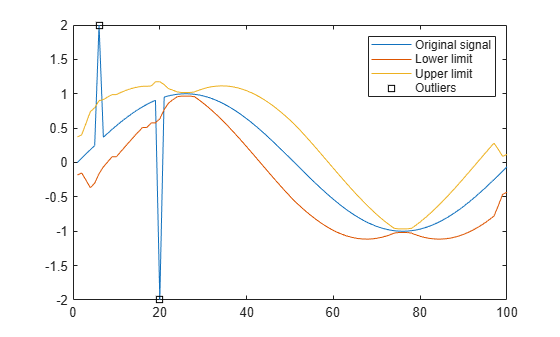Repeat the computation, but now take just one adjacent sample on each side when computing the median. The function considers the extrema as outliers.

hampel(x,1)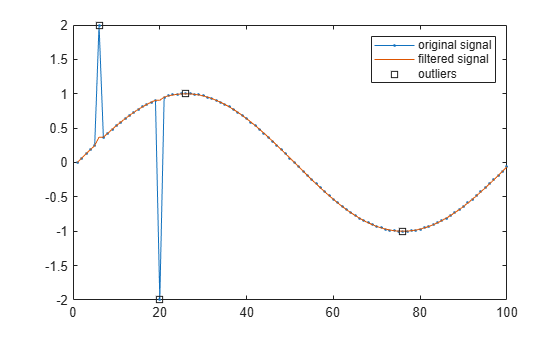Generate a two-channel signal consisting of sinusoids of different frequencies. Place spikes in random places. Use NaNs to add missing samples at random. Reset the random number generator for reproducible results. Plot the signal.

rng('default')

n = 59;
x = sin(pi./[15 10]'*(1:n)+pi/3)';

spk = randi(2*n,9,1);
x(spk) = x(spk)*2;
x(randi(2*n,6,1)) = NaN;

plot(x)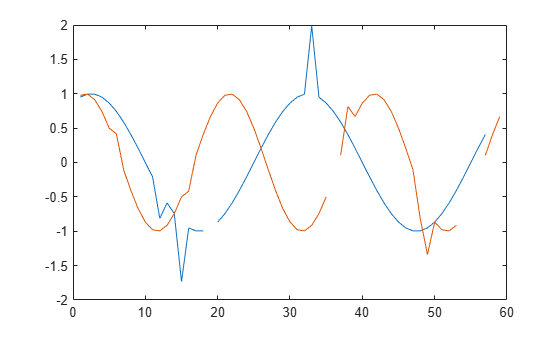Filter the signal using hampel with the default settings.

y = hampel(x);
plot(y)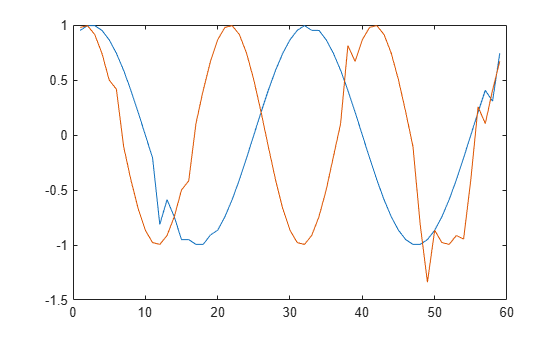Increase the length of the moving window and decrease the threshold to treat a sample as an outlier.

y = hampel(x,4,2);
plot(y)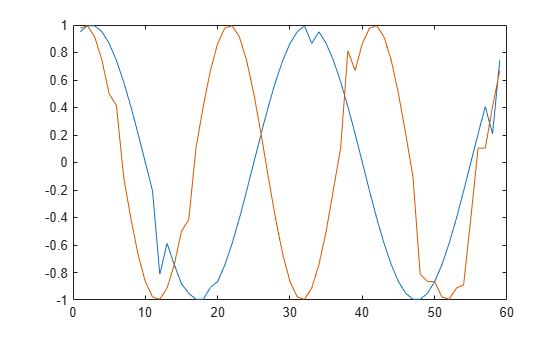Output the running median for each channel. Overlay the medians on a plot of the signal.

[y,j,xmd,xsd] = hampel(x,4,2);
plot(x)
hold on
plot(xmd,'--')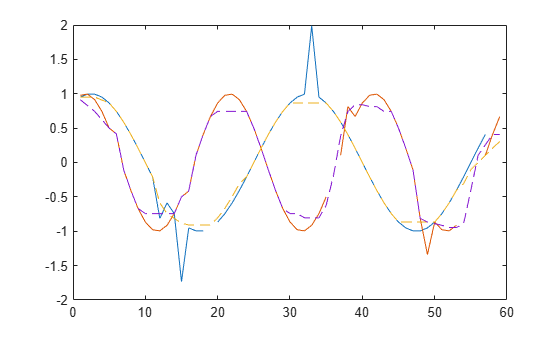Generate a multichannel signal that consists of two sinusoids of different frequencies embedded in white Gaussian noise of unit variance.

rng('default')

t = 0:60;
x = sin(pi./[10;2]*t)'+randn(numel(t),2);

Apply a Hampel filter to the signal. Take as outliers those points that differ by more than two standard deviations from the median of a surrounding nine-sample window. Output a logical matrix that is true at the locations of the outliers.

k = 4;
nsig = 2;

[y,h] = hampel(x,k,nsig);

Plot each channel of the signal in its own set of axes. Draw the original signal, the filtered signal, and the outliers. Annotate the outlier locations.

for k = 1:2
hk = h(:,k);
ax = subplot(2,1,k);
plot(t,x(:,k))
hold on
plot(t,y(:,k))
plot(t(hk),x(hk,k),'*')
hold off
ax.XTick = t(hk);
endGenerate 100 samples of a sinusoidal signal. Replace the sixth and twentieth samples with spikes.

n = 1:100;
x = sin(2*pi*n/100);
x(6) = 2;
x(20) = -2;

Use hampel to compute the local median and estimated standard deviation for every sample. Use the default values of the input parameters:

• The window size is $2×3+1=7$.

• The points that differ from their window median by more than three standard deviations are considered outliers.

Plot the result.

[y,i,xmedian,xsigma] = hampel(x);

plot(n,x)
hold on
plot(n,[1;1]*xmedian+3*[-1;1]*xsigma)
plot(find(i),x(i),'sk')
hold off
legend('Signal','Lower','Upper','Outliers')Repeat the calculation using a window size of $2×10+1=21$ and two standard deviations as the criteria for identifying outliers.

sds = 2;

plot(n,x)
hold on
plot(n,[1;1]*xmedian+sds*[-1;1]*xsigma)
plot(find(i),x(i),'sk')
hold off
legend('Signal','Lower','Upper','Outliers')Input Arguments

collapse all

Input signal, specified as a vector or matrix. If x is a matrix, then hampel treats each column of x as an independent channel.

Example: cos(pi/4*(0:159))+randn(1,160) is a single-channel row-vector signal.

Example: cos(pi./[4;2]*(0:159))'+randn(160,2) is a two-channel signal.

Data Types: single | double

Number of neighbors on either side of the sample xs, specified as an integer scalar. Samples close to the signal edges that have fewer than k samples on one side are compared to the median of a smaller window.Data Types: single | double

Number of standard deviations by which a sample of x must differ from its local median to be considered an outlier. Specify nsigma as a real scalar. The function estimates the standard deviation by scaling the local median absolute deviation (MAD) by a factor of $\kappa =\frac{1}{\sqrt{2}{\mathrm{erf}}^{-1}\left(1/2\right)}\approx 1.4826$.

Data Types: single | double

Output Arguments

collapse all

Filtered signal, returned as a vector or matrix of the same size as x.

Data Types: single | double

Outlier index, returned as a vector or matrix of the same size as x.

Data Types: logical

Local medians, returned as a vector or matrix of the same size as x.

Data Types: single | double

Estimated standard deviations, returned as a vector or matrix of the same size as x.

Data Types: single | double

collapse all

Hampel Identifier

The Hampel identifier is a variation of the three-sigma rule of statistics that is robust against outliers.

Given a sequence x1, x2, x3, …, xn and a sliding window of length k, define point-to-point median and standard-deviation estimates using:

• Local median — ${m}_{i}=\mathrm{median}\left({x}_{i-k},{x}_{i-k+1},{x}_{i-k+2},\dots ,{x}_{i},\dots ,{x}_{i+k-2},{x}_{i+k-1},{x}_{i+k}\right)$

• Standard deviation — ${\sigma }_{i}=\kappa \mathrm{median}\left(|{x}_{i-k}-{m}_{i}|,\dots ,|{x}_{i+k}-{m}_{i}|\right),$ where $\kappa =\frac{1}{\sqrt{2}{\mathrm{erf}}^{-1}\left(1/2\right)}\approx 1.4826$

The quantity σi /κ is known as the median absolute deviation (MAD).

If a sample xi is such that

$|{x}_{i}-{m}_{i}|>{n}_{\sigma }{\sigma }_{i}$

for a given threshold nσ, then the Hampel identifier declares xi an outlier and replaces it with mi.

Near the sequence endpoints, the function truncates the window used to compute mi and σi:

• i < k + 1

${m}_{i}=\mathrm{median}\left({x}_{1},{x}_{2},{x}_{3},\dots ,{x}_{i},\dots ,{x}_{i+k-2},{x}_{i+k-1},{x}_{i+k}\right)$

${\sigma }_{i}=\kappa \mathrm{median}\left(|{x}_{1}-{m}_{1}|,\dots ,|{x}_{i+k}-{m}_{i}|\right)$

• i > nk

${m}_{i}=\mathrm{median}\left({x}_{i-k},{x}_{i-k+1},{x}_{i-k+2},\dots ,{x}_{i},\dots ,{x}_{n-2},{x}_{n-1},{x}_{n}\right)$

${\sigma }_{i}=\kappa \mathrm{median}\left(|{x}_{i-k}-{m}_{i}|,\dots ,|{x}_{n}-{m}_{n}|\right)$

 Liu, Hancong, Sirish Shah, and Wei Jiang. “On-line outlier detection and data cleaning.” Computers and Chemical Engineering. Vol. 28, March 2004, pp. 1635–1647.